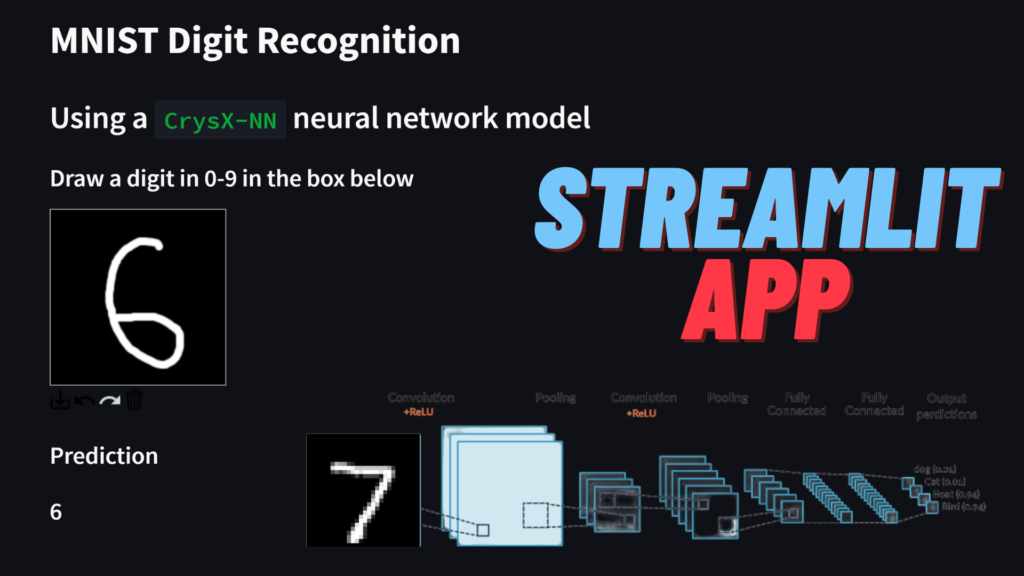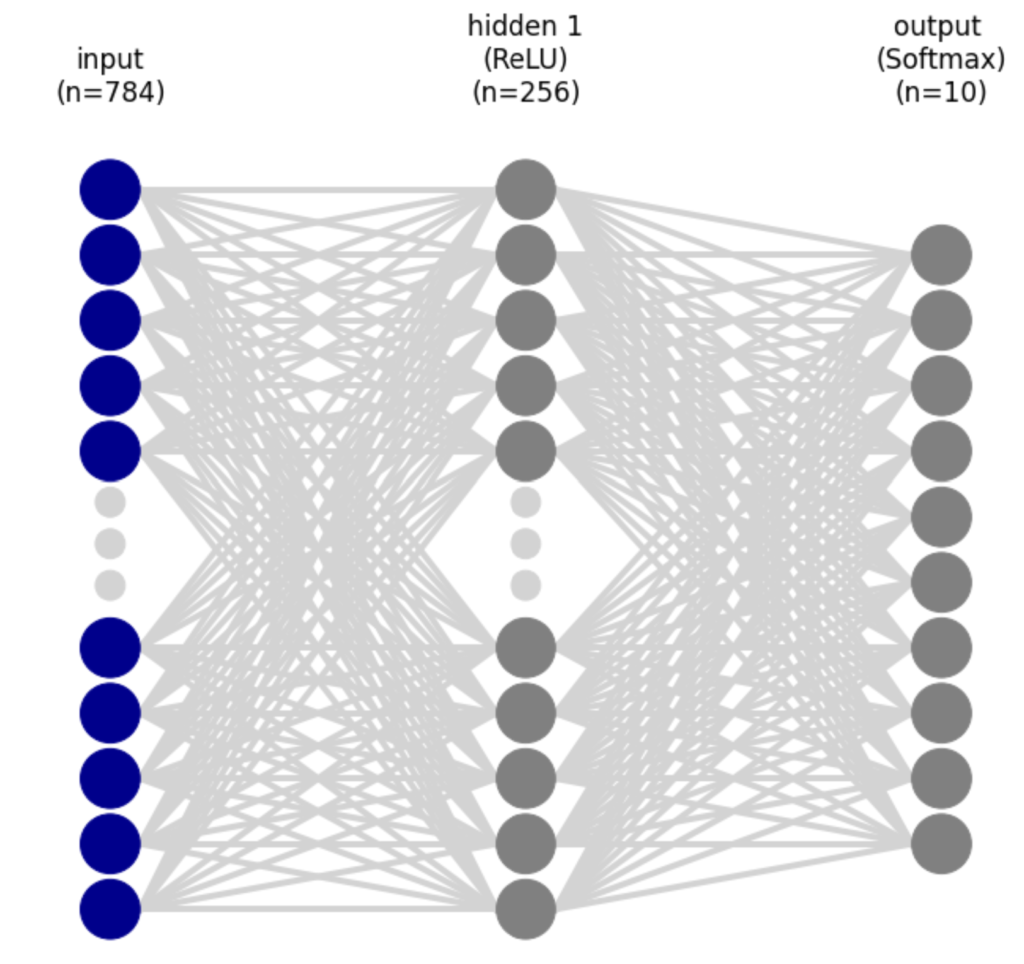# Handwritten digit recognition using CrysX – Neural Network library and Streamlit Web AppRecently, I wrote a blog post on creating a Streamlit app for recognizing handwritten digits using a convolutional neural network model with the help of the PyTorch library.

It worked quite well as expected.

However, recently I had also published my own Neural Network Python library called CrysX-NN (crysx_nn). It is extremely efficient and can make use of both CPU and GPU. Moreover, it is written in pure python using Numpy, Cupy and Numba for simplicity and efficiency.

So, I decided to see how well can a plain neural network perform against a convolutional neural network. We would expect the CNN to be better of course. But just how better is it?

To answer this question, I trained a Neural Network model on the MNIST dataset and tested its performance on real-world data using a Streamlit web app that lets the user enter their own handwritten digits using mouse/touch input. The neural network has an input layer of size 784 (28×28 pixels = 784 pixels), 1 hidden layer of size 256 with the ReLU activation function, and finally an output layer of size 10 (for 0-9 digits) with the Softmax activation function to get the probabilities for each digit.In the streamlit app below you can write a digit using the mouse/touch input. The details of the model and the app are given below the demo.

### Streamlit App

https://share.streamlit.io/manassharma07/mnist-plus/main/mnist_NN_crysx_app.py

The app above is quite neat and also shows what the prediction process looks like.

The user’s handwritten input is captured in a 200×200 pixel box, which is then converted to an image. Subsequently, image processing is done to find a rectangle that completely encompasses the digit blob. Then this rectangular crop of the user input is further processed. Firstly it is converted to grayscale, then resized to a 22×22 image using BILINEAR interpolation. Then a padding of 3 pixels is applied on all the sides of the image to get a 28×28 image. This image still has pixel values in the range of 0-255. These are then normalized by dividing by 255. Then the pixel values are standardized by using the mean and standard deviation of the training MNIST dataset. Finally, the processed image is passed to the CNN model to make a prediction.

You can notice how the predictions are wrong a lot of times. Therefore, compared to the app based on CNN which was only problematic for the digits 1 and 7, this is a lot worse.

### Source code for the app

https://github.com/manassharma07/MNIST-PLUS/blob/main/mnist_NN_crysx_app.py

```from streamlit_drawable_canvas import st_canvas
import streamlit as st
import matplotlib.pyplot as plt
import numpy as np
from PIL import Image
import cv2
from crysx_nn import network

@st.cache
nInputs = 784 # No. of nodes in the input layer
neurons_per_layer = [256, 10] # Neurons per layer (excluding the input layer)
activation_func_names = ['ReLU', 'Softmax']
nLayers = len(neurons_per_layer)
batchSize = 32 # No. of input samples to process at a time for optimization
# Create the crysx_nn neural network model
model = network.nn_model(nInputs=nInputs, neurons_per_layer=neurons_per_layer, activation_func_names=activation_func_names, batch_size=batchSize, device='CPU', init_method='Xavier')
# Load the preoptimized weights and biases
return model

st.write('# MNIST Digit Recognition')
st.write('## Using a `CrysX-NN` neural network model')

st.write('### Draw a digit in 0-9 in the box below')
# Specify canvas parameters in application
stroke_width = st.sidebar.slider("Stroke width: ", 1, 25, 9)

realtime_update = st.sidebar.checkbox("Update in realtime", True)

# @st.cache
def make_sidebar():
# st.sidebar.markdown("## [CrysX-NN](https://github.com/manassharma07/crysx_nn)")
st.sidebar.write('\n\n ## Neural Network Library Used')
# st.sidebar.image('logo_crysx_nn.png')
st.sidebar.caption('https://github.com/manassharma07/crysx_nn')
st.sidebar.write('## Neural Network Architecture Used')
st.sidebar.write('1. **Inputs**: Flattened 28x28=784')
st.sidebar.write('2. **Hidden layer** of size **256** with **ReLU** activation Function')
st.sidebar.write('3. **Output layer** of size **10** with **Softmax** activation Function')
st.sidebar.write('Training was done for 10 epochs with Binary Cross Entropy Loss function and a batch size of 32.')
# st.sidebar.image('neural_network_visualization.png')

make_sidebar()

# Create a canvas component
canvas_result = st_canvas(
fill_color="rgba(255, 165, 0, 0.3)",  # Fixed fill color with some opacity
stroke_width=stroke_width,
stroke_color='#FFFFFF',
background_color='#000000',
#background_image=Image.open(bg_image) if bg_image else None,
update_streamlit=realtime_update,
height=200,
width=200,
drawing_mode='freedraw',
key="canvas",
)

# Do something interesting with the image data and paths
if canvas_result.image_data is not None:

# st.write('### Image being used as input')
# st.image(canvas_result.image_data)
# st.write(type(canvas_result.image_data))
# st.write(canvas_result.image_data.shape)
# st.write(canvas_result.image_data)
# im = Image.fromarray(canvas_result.image_data.astype('uint8'), mode="RGBA")
# im.save("user_input.png", "PNG")

# Get the numpy array (4-channel RGBA 100,100,4)
input_numpy_array = np.array(canvas_result.image_data)

# Get the RGBA PIL image
input_image = Image.fromarray(input_numpy_array.astype('uint8'), 'RGBA')
input_image.save('user_input.png')

# Convert it to grayscale
input_image_gs = input_image.convert('L')
input_image_gs_np = np.asarray(input_image_gs.getdata()).reshape(200,200)
all_zeros = not np.any(input_image_gs_np)
if not all_zeros:
# st.write('### Image as a grayscale Numpy array')
# st.write(input_image_gs_np)

# Create a temporary image for opencv to read it
input_image_gs.save('temp_for_cv2.jpg')
# Start creating a bounding box
height, width = image.shape
x,y,w,h = cv2.boundingRect(image)

# Create new blank image and shift ROI to new coordinates
ROI = image[y:y+h, x:x+w]
#     print(ROI.shape)
x = width//2 - ROI.shape//2
y = height//2 - ROI.shape//2
#     print(x,y)
# Check if centering/masking was successful
# Now we need to resize, but it causes problems with default arguments as it changes the range of pixel values to be negative or positive
# compressed_output_image = output_image.resize((22,22))
# Therefore, we use the following:
compressed_output_image = output_image.resize((22,22), Image.BILINEAR) # PIL.Image.NEAREST or PIL.Image.BILINEAR also performs good

tensor_image = np.array(compressed_output_image.getdata())/255.
tensor_image = tensor_image.reshape(22,22)
tensor_image = np.pad(tensor_image, (3,3), "constant", constant_values=(0,0))
# Normalization should be done after padding i guess
tensor_image = (tensor_image - 0.1307) / 0.3081
# st.write(tensor_image.shape)
# Shape of tensor image is (1,28,28)

# st.write('### Processing steps:')
# st.write('1. Find the bounding box of the digit blob and use that.')
# st.write('2. Convert it to size 22x22.')
# st.write('3. Pad the image with 3 pixels on all the sides to get a 28x28 image.')
# st.write('4. Normalize the image to have pixel values between 0 and 1.')
# st.write('5. Standardize the image using the mean and standard deviation of the MNIST_plus dataset.')

# The following gives noisy image because the values are from -1 to 1, which is not a proper image format
# im = Image.fromarray(tensor_image.reshape(28,28), mode='L')
# im.save("processed_tensor.png", "PNG")
# So we use matplotlib to save it instead
plt.imsave('processed_tensor.png',tensor_image.reshape(28,28), cmap='gray')

# st.write('### Processed image')
# st.image('processed_tensor.png')
# st.write(tensor_image.detach().cpu().numpy().reshape(28,28))

### Compute the predictions
output_probabilities = model.predict(tensor_image.reshape(1,784).astype(np.float32))
prediction = np.argmax(output_probabilities)

top_3_probabilities = output_probabilities.argsort()[-3:][::-1]
ind = output_probabilities.argsort()[-3:][::-1]
top_3_certainties = output_probabilities[0,ind]*100

st.write('### Prediction')
st.write('### '+str(prediction))

st.write('Original MNIST Dataset available as PNGs at: https://github.com/manassharma07/MNIST-PLUS/tree/main/mnist_orig_png')

st.write('## Breakdown of the prediction process:')

st.write('### Image being used as input')
st.image(canvas_result.image_data)

st.write('### Image as a grayscale Numpy array')
st.write(input_image_gs_np)

st.write('### Processing steps:')
st.write('1. Find the bounding box of the digit blob and use that.')
st.write('2. Convert it to size 22x22.')
st.write('3. Pad the image with 3 pixels on all the sides to get a 28x28 image.')
st.write('4. Normalize the image to have pixel values between 0 and 1.')
st.write('5. Standardize the image using the mean and standard deviation of the MNIST training dataset.')

st.write('### Processed image')
st.image('processed_tensor.png')

# st.write('### Processed Image as a grayscale Numpy array')
# st.write(tensor_image.reshape(28,28))

st.write('### Prediction')
st.write(str(prediction))
st.write('### Certainty')
st.write(str(output_probabilities[0,prediction]*100) +'%')
st.write('### Top 3 candidates')
# st.write(top_3_probabilities)
st.write(str(top_3_probabilities))
st.write('### Certainties %')
# st.write(top_3_certainties)
st.write(str(top_3_certainties))

```

### Pretrained Neural Network weights and biases for the CrysX-NN model

```model.load_model_weights('NN_crysx_mnist_98.11_weights')
```

### Code used for training the model

https://github.com/manassharma07/crysx_nn/blob/main/examples/NN_MNIST_orig_from_raw_png_crysx.ipynb

### Details of the Neural Network

Learning Rate = 0.3
Number of epochs = 10
Batch size = 200
Loss function: Categorical Cross Entropy loss

#### Code snippet for creation and training of Neural network

```    from crysx_nn import mnist_utils as mu
import numpy as np

## Load the training dataset from MNIST_orig in memory (May take upto 5 min)
path = 'MNIST-PLUS-PNG/mnist_orig_png'
trainData, trainLabels = mu.loadMNIST(path_main=path, train=True, shuffle=True)

## Normalize within the range [0,1.0]
trainData = trainData/255 # Normalize

trainData_mean = trainData.mean()
trainData_std = trainData.std()

## Standardize the data so that it has mean 0 and variance 1
trainData = (trainData - np.mean(trainData)) / np.std(trainData)

## Convert labels to one-hot vectors
trainLabels = mu.one_hot_encode(trainLabels, 10)

## Flatten the input numpy arrays (nSamples,28,28)->(nSamples, 784)
trainData = trainData.reshape(trainData.shape, 784)

## Let us create a NN using CrysX-NN now
nInputs = 784 # No. of nodes in the input layer
neurons_per_layer = [256, 10] # Neurons per layer (excluding the input layer)
activation_func_names = ['ReLU', 'Softmax']
nLayers = len(neurons_per_layer)
nEpochs = 10
batchSize = 200 # No. of input samples to process at a time for optimization

from crysx_nn import network
model = network.nn_model(nInputs=nInputs, neurons_per_layer=neurons_per_layer, activation_func_names=activation_func_names, batch_size=batchSize, device='CPU', init_method='Xavier')

model.lr = 0.3

## Check the model details
model.details()
model.visualize()

## Optimize/Train the network
inputs = trainData.astype(np.float32)
outputs = trainLabels.astype(np.float32)
# Run optimization
# model.optimize(inputs, outputs, lr=0.3,nEpochs=nEpochs,loss_func_name='CCE', miniterEpoch=1, batchProgressBar=True, miniterBatch=100)
# To get accuracies at each epoch
model.optimize(inputs, outputs, lr=0.3,nEpochs=nEpochs,loss_func_name='CCE', miniterEpoch=1, batchProgressBar=True, miniterBatch=100, get_accuracy=True)

## Error at each epoch
print(model.errors)

## Accuracy at each epoch
print(model.accuracy)

## Save model weights and biases
# Save weights
model.save_model_weights('NN_crysx_mnist_98.11_weights')
# Save biases
model.save_model_biases('NN_crysx_mnist_98.11_biases')

## Load model weights and biases from files

## Test data set
path = 'MNIST-PLUS-PNG/mnist_orig_png'
testData, testLabels = mu.loadMNIST(path_main=path, train=False, shuffle=True)

## Normalize within the range [0,1.0]

testData = testData/255. # Normalize

## Standardize the data so that it has mean 0 and variance 1
# Use the mean and std of training data **********
testData = (testData - trainData_mean) / trainData_std

## Convert labels to one-hot vectors
testLabels = mu.one_hot_encode(testLabels, 10)

## Flatten the input numpy arrays (nSamples,28,28)->(nSamples, 784)
testData = testData.reshape(testData.shape, 784)

## Performance on Test data
# Convert to float32 arrays
inputs = testData.astype(np.float32)
outputs = testLabels.astype(np.float32)

predictions, error, accuracy = model.predict(inputs, outputs, loss_func_name='CCE', get_accuracy=True)
print('Error:',error)
print('Accuracy %:',accuracy*100)
```[wpedon id="7041" align="center"]